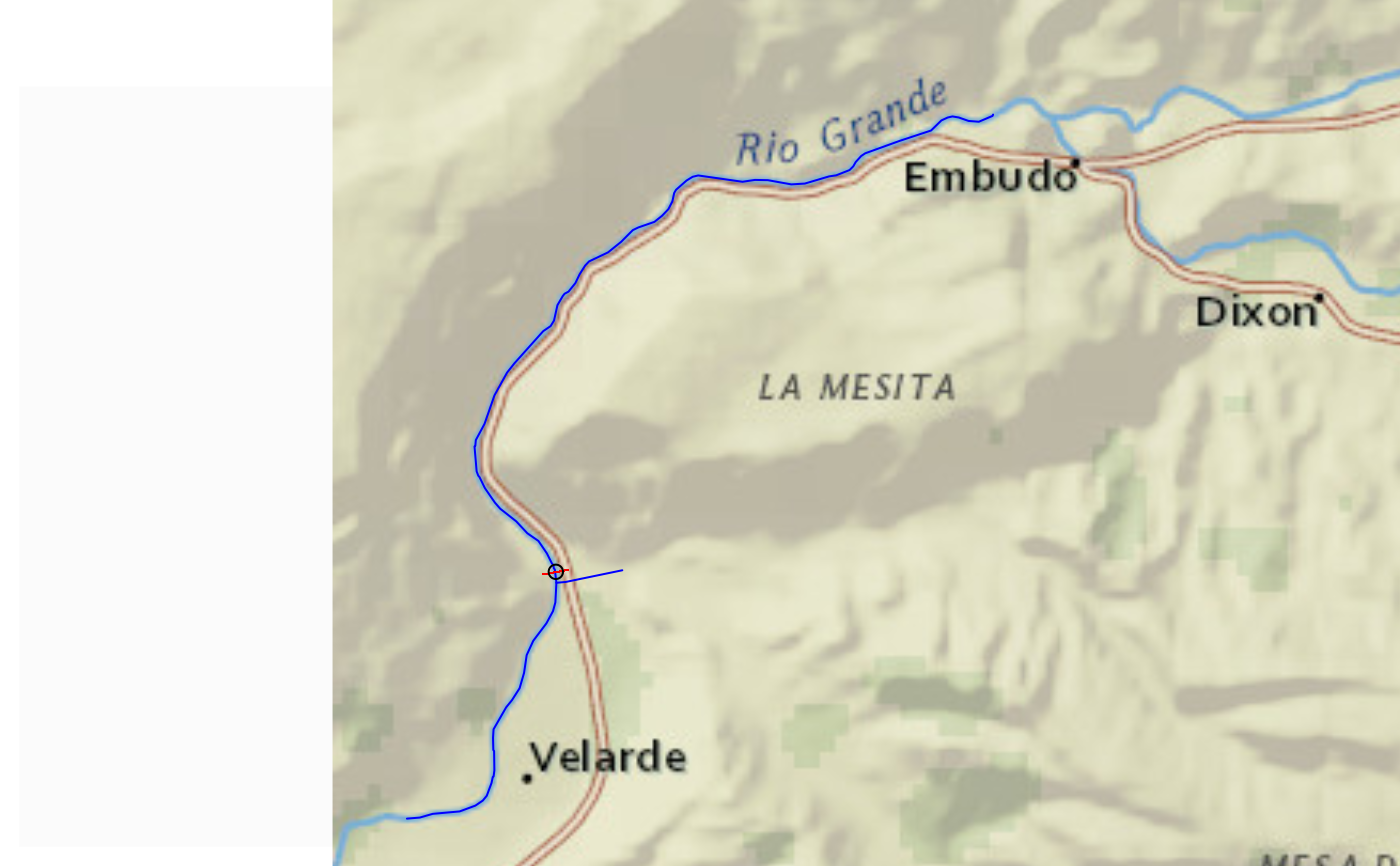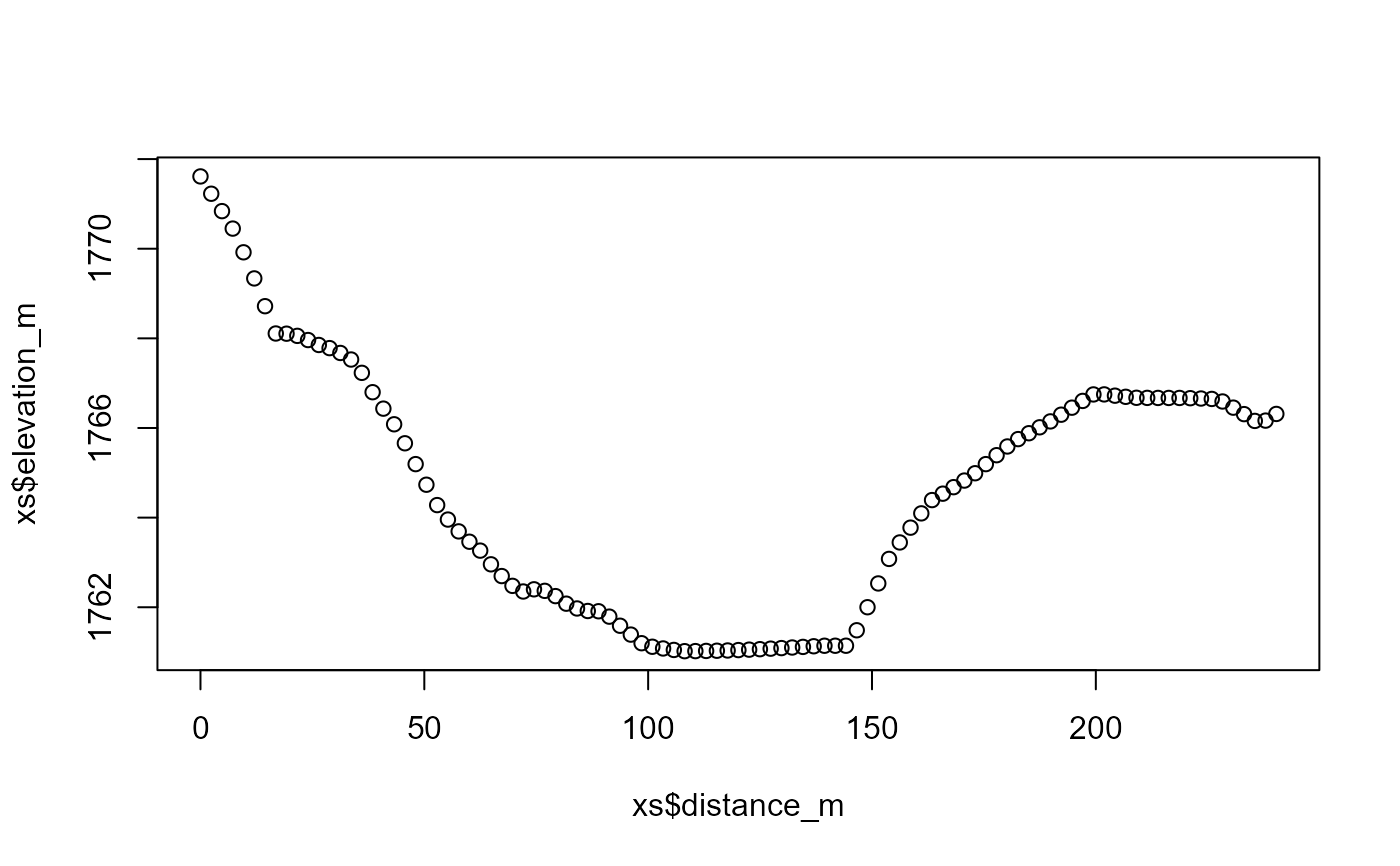Uses a cross section retrieval web services to retrieve a cross section given a point and specified width. Orientation is determined based on direction of a the flowline found near point. This function uses a 10m National Elevation Dataset request on the back end.

get_xs_point(point, width, num_pts)

## Arguments

point

sfc POINT including crs as created by: sf::st_sfc(sf::st_point(.. ,..), crs)crs.

width

Cross section width in meters.

num_pts

numeric number of points to retrieve along the cross section.

## Value

sf data.frame containing points retrieved.

## Examples

# \donttest{
point <- sf::st_sfc(sf::st_point(x = c(-105.97218, 36.17592)), crs = 4326)

(xs <- get_xs_point(point, 300, 100))
#> Simple feature collection with 101 features and 4 fields
#> Geometry type: POINT
#> Dimension:     XY
#> Bounding box:  xmin: -105.9735 ymin: 36.1757 xmax: -105.9709 ymax: 36.17609
#> Geodetic CRS:  WGS 84
#> # A tibble: 101 × 5
#>    id    spatial_ref elevation_m distance_m             geometry
#>    <chr>       <int>       <dbl>      <dbl>          <POINT [°]>
#>  1 0               0       1772.       0     (-105.9735 36.1757)
#>  2 1               0       1771.       2.40  (-105.9735 36.1757)
#>  3 2               0       1771.       4.81  (-105.9735 36.1757)
#>  4 3               0       1770.       7.21 (-105.9734 36.17571)
#>  5 4               0       1770.       9.61 (-105.9734 36.17571)
#>  6 5               0       1769.      12.0  (-105.9734 36.17572)
#>  7 6               0       1769.      14.4  (-105.9734 36.17572)
#>  8 7               0       1769.      16.8  (-105.9733 36.17572)
#>  9 8               0       1768.      19.2  (-105.9733 36.17573)
#> 10 9               0       1768.      21.6  (-105.9733 36.17573)
#> # ℹ 91 more rows

if(inherits(xs, "sf")) {

bbox <- sf::st_bbox(xs) + c(-0.005, -0.005, 0.005, 0.005)

nhdplusTools::plot_nhdplus(bbox = bbox, cache_data = FALSE)

plot(sf::st_transform(sf::st_geometry(xs), 3857), pch = ".", add = TRUE, col = "red")
plot(sf::st_transform(sf::st_sfc(point, crs = 4326), 3857), add = TRUE)

plot(xs$distance_m, xs$elevation_m)
}
#> Spherical geometry (s2) switched off
#> Found invalid geometry, attempting to fix.
#> although coordinates are longitude/latitude, st_intersects assumes that they
#> are planar
#> Spherical geometry (s2) switched on
#> Spherical geometry (s2) switched off
#> although coordinates are longitude/latitude, st_intersects assumes that they
#> are planar
#> Spherical geometry (s2) switched on
#> Spherical geometry (s2) switched off
#> Warning: No nhdarea features found
#> Spherical geometry (s2) switched on
#> Spherical geometry (s2) switched off
#> Warning: No waterbodies features found
#> Spherical geometry (s2) switched on
#> Spherical geometry (s2) switched off
#> Warning: No nonnetwork features found
#> Spherical geometry (s2) switched on
#> Zoom set to: 12# }